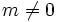# Isomorph-automorphic subgroup

## Contents

BEWARE! This term is nonstandard and is being used locally within the wiki. [SHOW MORE]
This article defines a subgroup property: a property that can be evaluated to true/false given a group and a subgroup thereof, invariant under subgroup equivalence. View a complete list of subgroup properties[SHOW MORE]

## Definition

### Symbol-free definition

A subgroup of a group is termed isomorph-automorphic if given any other isomorphic subgroup, there is an automorphism of the whole group, mapping the subgroup isomorphically to the other one.

### Definition with symbols

A subgroup$H$ of a group$G$ is termed isomorph-automorphic if whenever there exists a subgroup$K$ of$G$ such that$H$ and$K$ are isomorphic groups, there exists an automorphism$\sigma$ of$G$ such that$\sigma(H) = K$.

## Examples

In a finite cyclic group, every subgroup is isomorph-automorphic (in fact, every subgroup is isomorph-free: no two subgroups are isomorphic).

Similarly, in a finite elementary Abelian group, every subgroup is isomorph-automorphic. That's because given any two subspaces of a finite-dimensional vector space that have the same dimension, there is an automorphism of the whole space taking one to the other.

By Sylow's theorem, every Sylow subgroup is isomorph-automorphic.

On the other hand, many subgroups are not isomorph-automorphic:

1. In$\mathbb{Z}$, the group of integers, any nontrivial subgroup is of the form$m\mathbb{Z}$,$m \ne 0$, hence is isomorphic to$\mathbb{Z}$. However, there is clearly no automorphism of$\mathbb{Z}$ mapping it to a proper subgroup.
2. Any infinite-dimensional vector space is isomorphic to a subspace of codimension one, but there is no automorphism mapping the whole space to such a subspace.
3. In the direct product$\mathbb{Z}/2\mathbb{Z} \times \mathbb{Z}/4\mathbb{Z}$, the direct factor$\mathbb{Z}/2\mathbb{Z}$ is isomorphic to the subgroup of multiples of 2 in the direct factor$\mathbb{Z}/4\mathbb{Z}$. However, there is no automorphism taking the first to the second.
4. In the dihedral group of order eight, the center is of order two, and any subgroup generated by a reflection has order two, so they are isomorphic. However, there is no automorphism taking the center to a subgroup generated by a reflection.